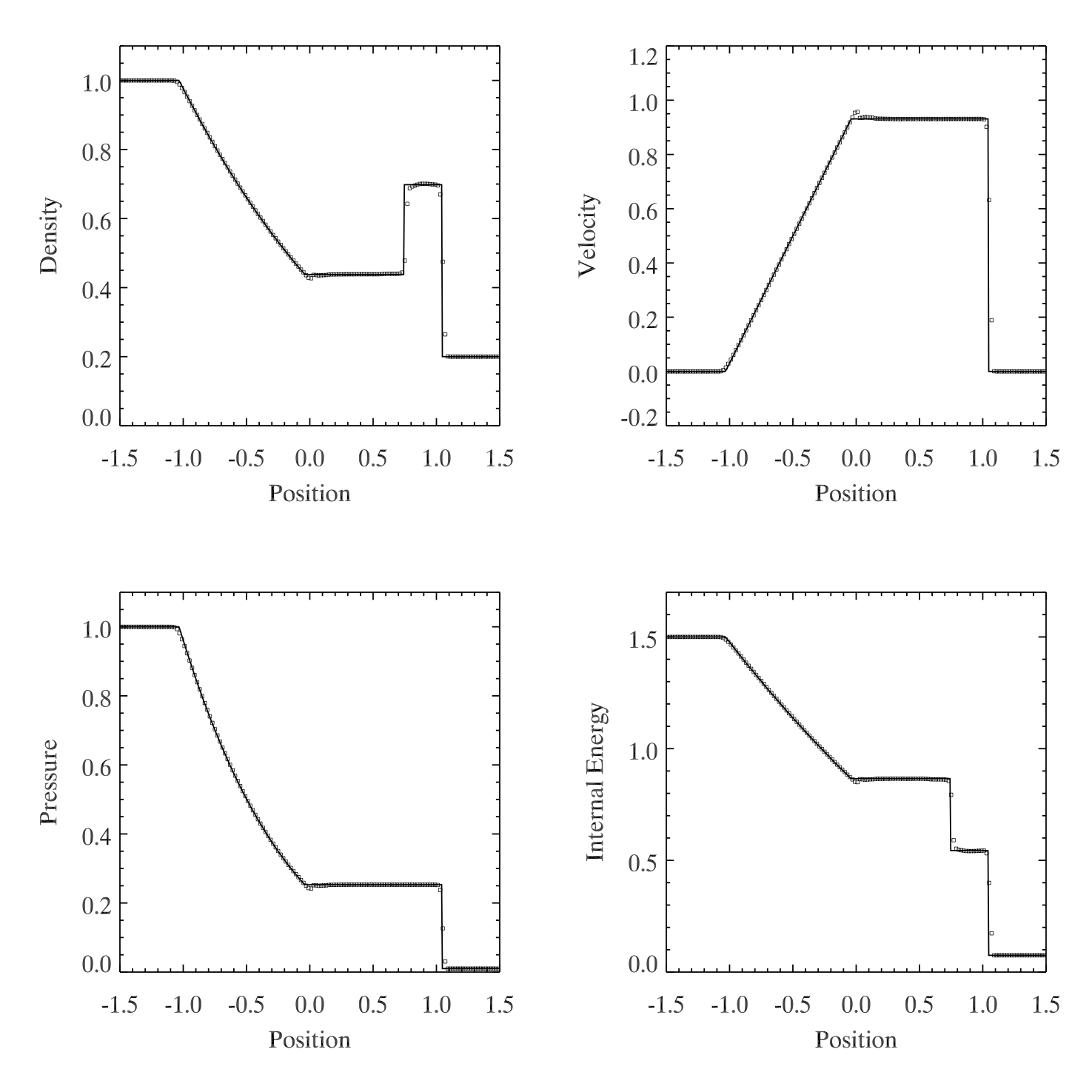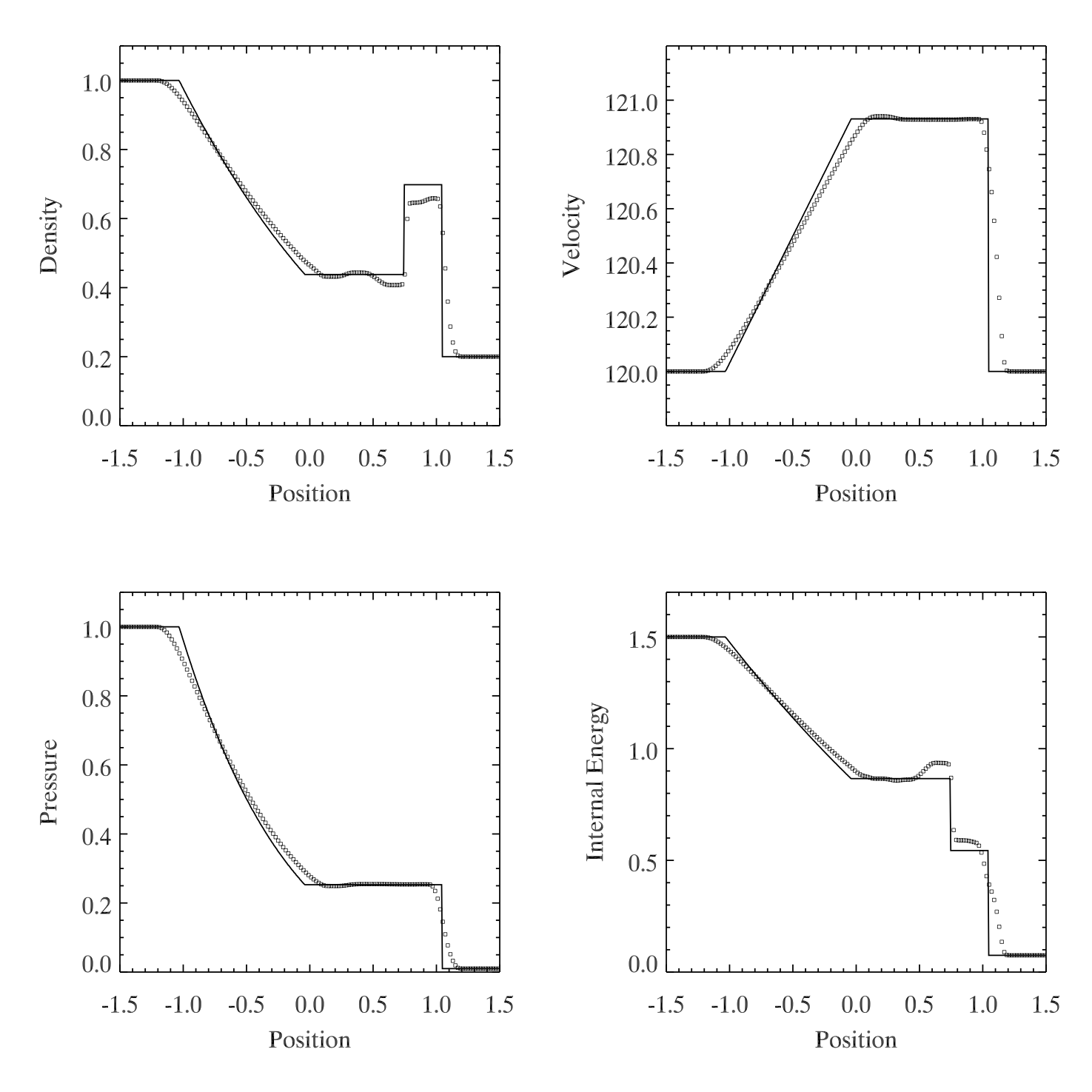Dual Energy Tests (Part 1)

September 29, 2015

Internal energy equations

From Teyssier 2015, the nonconservative internal energy update (Equation 161):

From Bryan et al. 2014, the nonconservative internal energy update (Equation 43):

The switch

We need to decide when to use the nonconservative internal energy update, instead of the conservatively calculated internal energy, $e_{\mathrm{int}} = e_T - 0.5\mathbf{v}\cdot\mathbf{v}.$

Note: in Cholla notation, $E = \rho e_T$, so $e_{\mathrm{int}} = \frac{p}{\rho (\gamma - 1.0)}$. Cholla tracks $E$ and $\rho e_{\mathrm{int}}$.

In Teyssier 2015, the switch is calculated using an estimate for the truncation error of the method.

In Bryan 2014, the switch is made according to the ratio of internal energy to total energy.

The tests

The tests shown below consist of a shock tube with periodic boundaries, to allow for high mach number bulk flow.

For the high mach number test, $v_L = v_R = 120$.

Without Dual Energy

Figure 1: PPMP, exact Riemann solver, CTUFigure 2: same as Figure 1, but with bulk velocity $v_{bulk} = 100 c_s$